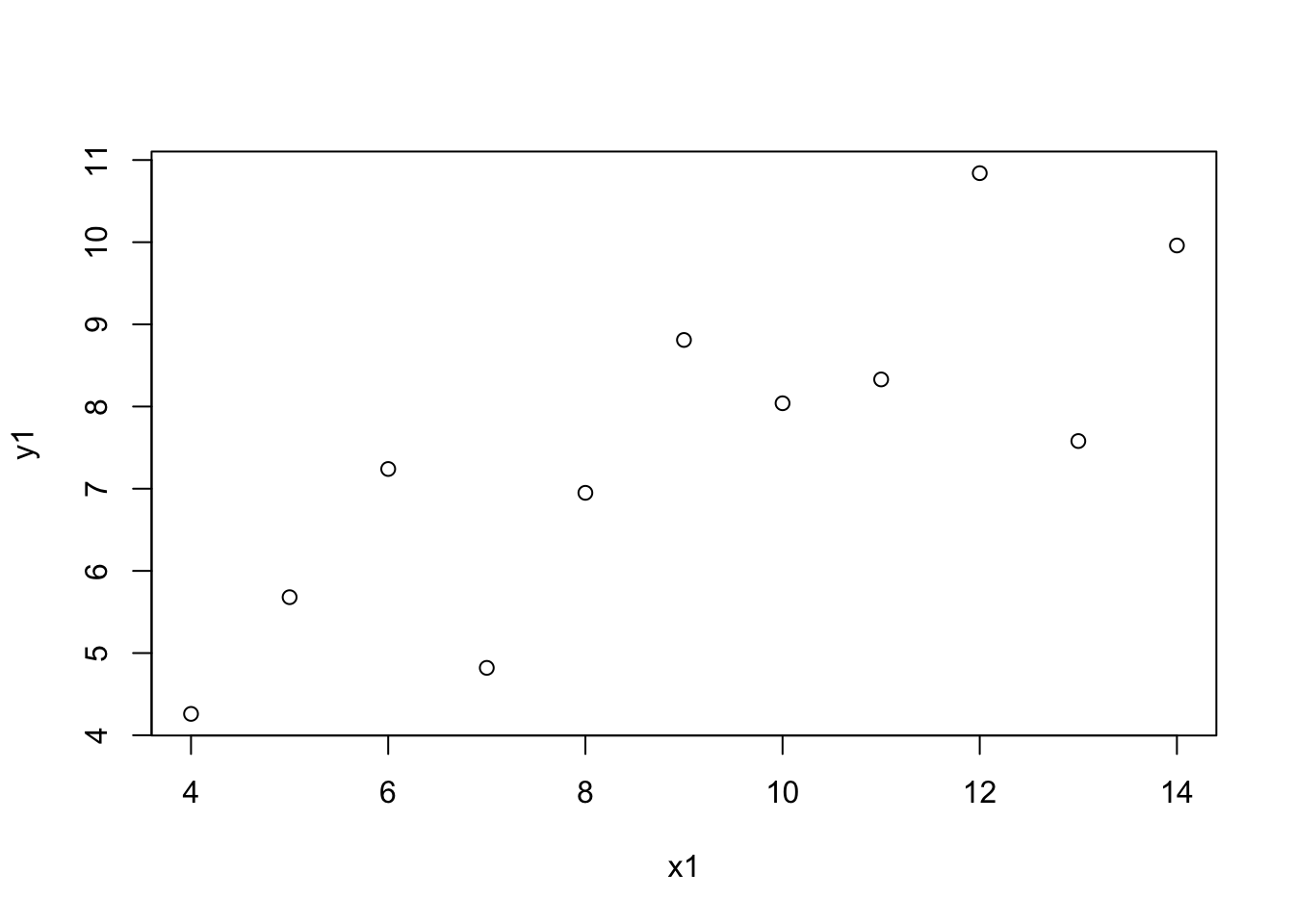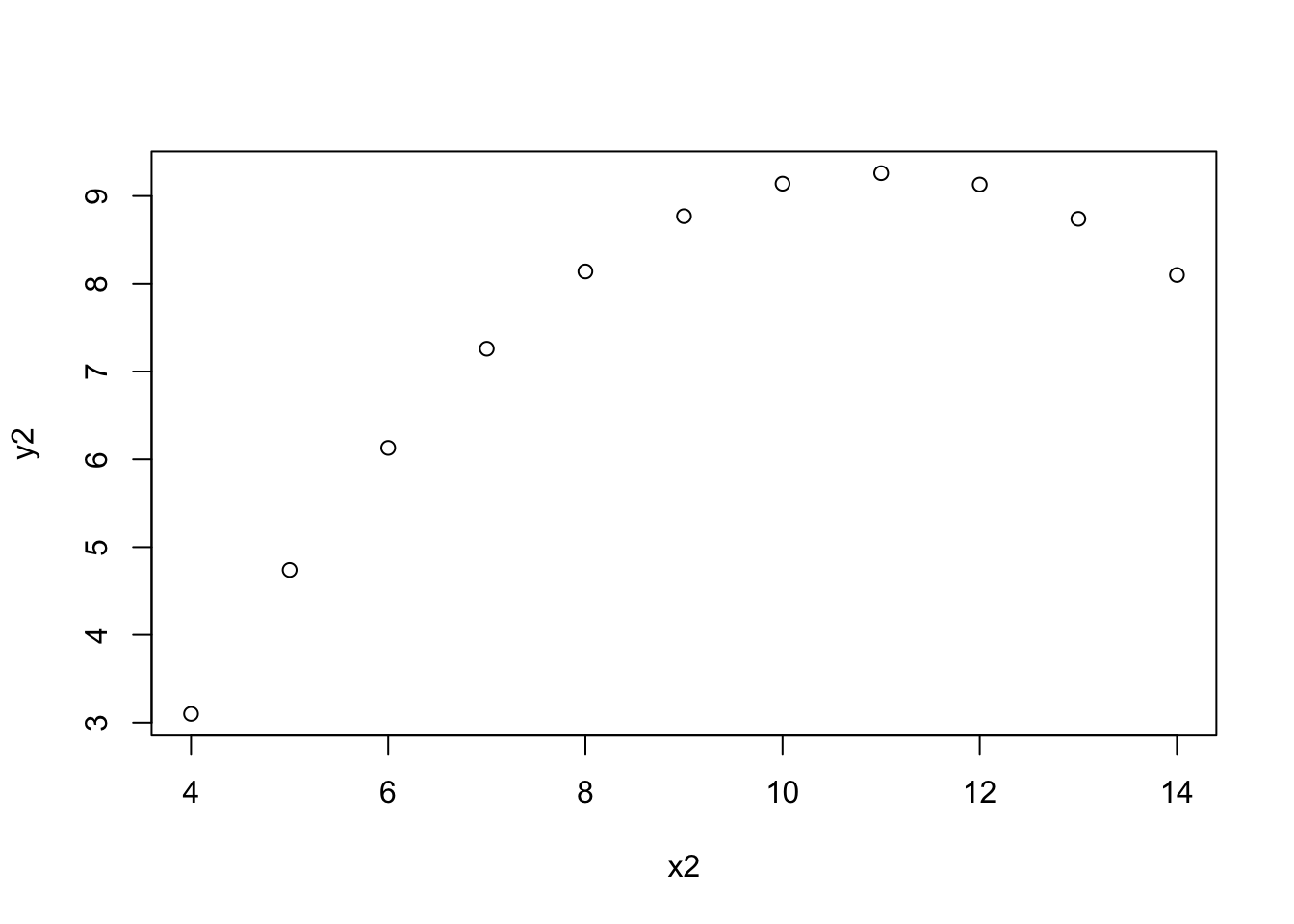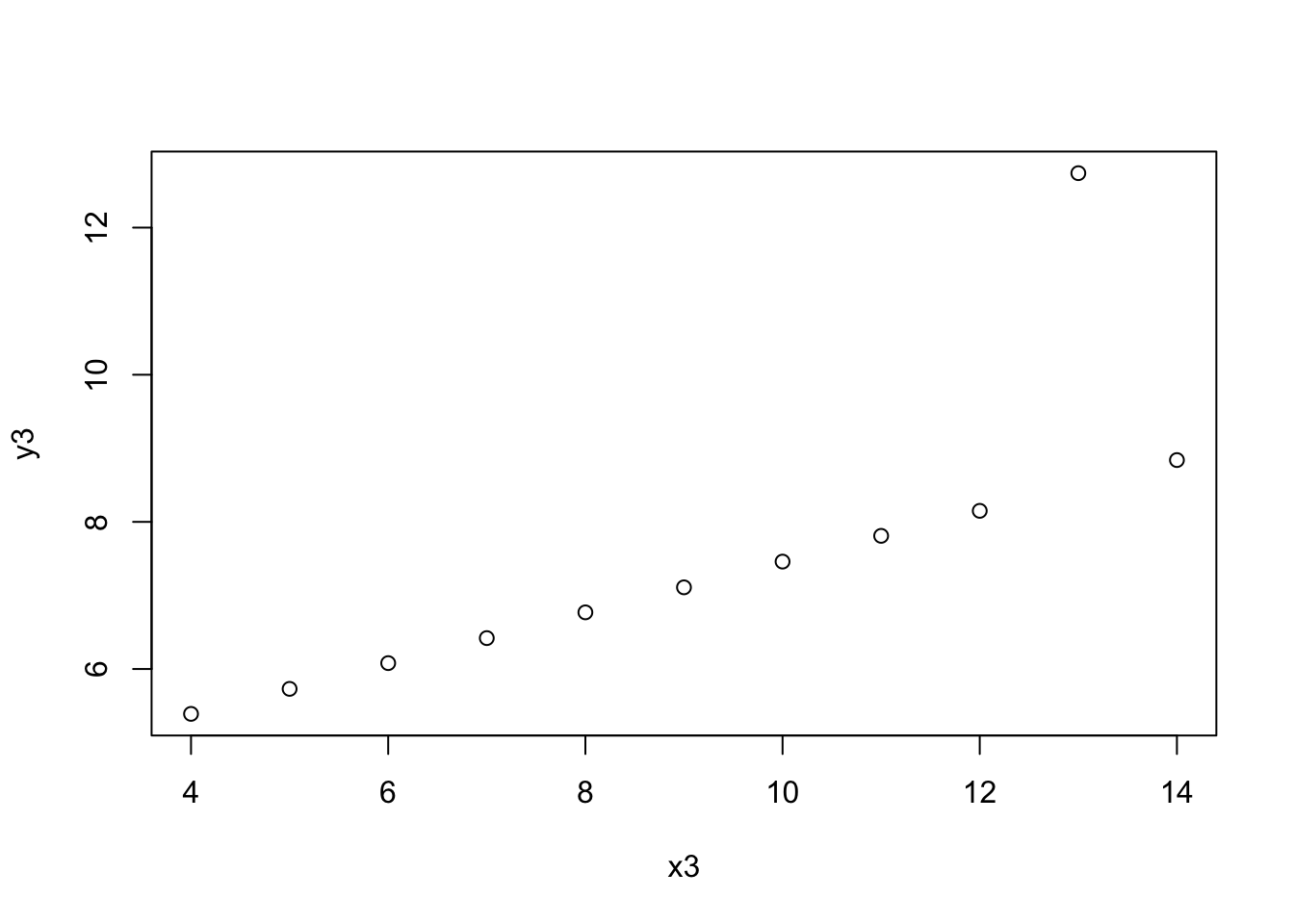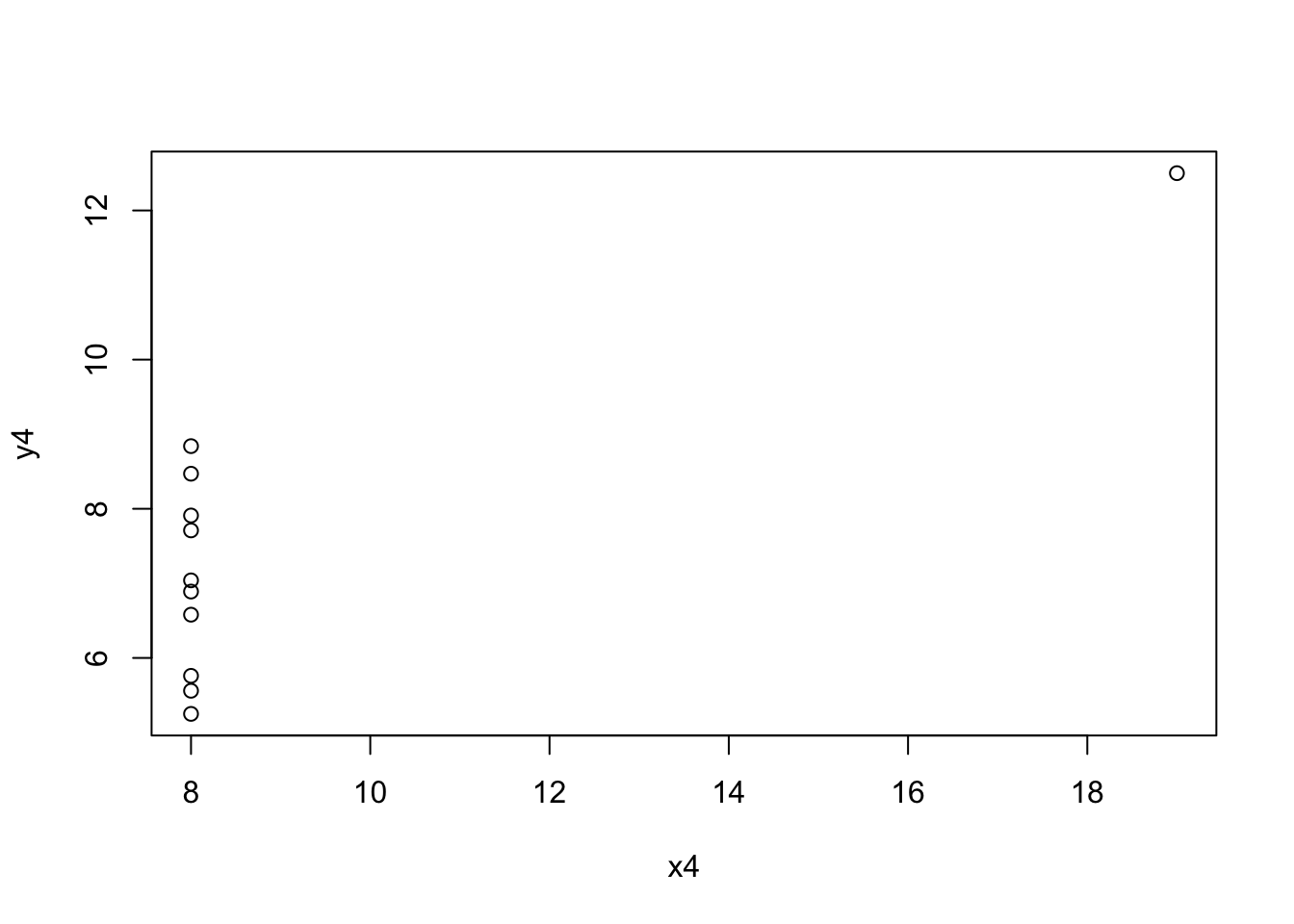# Thanks, Mike

R
visualization
mentors
Published

September 8, 2023

One stats class was required in my grad program, and Mike Meyer was my professor. The class was in S-plus, the closed source inspiration for R. And Mike regularly exhorted us (in his inimitable Australian accent), “Plot your data!”

Mike’s encouragment to use S-plus made it easier for me to adopt R when it appeared on the scene a few years later. Or at least I had fewer habits to change. And, Mike’s advice about plotting your data first always made sense to me. If you can’t see a pattern in the plots, how can you believe the inferential statistics?

In that spirit, mostly so that I can find it again, is an outstanding demonstration of that truth, the `datasauRus` package, based on Anscombe’s quartet , which is also available as the `anscombe` package.

``````library("ggplot2")
library("datasauRus")
ggplot(datasaurus_dozen, aes(x = x, y = y, colour = dataset))+
geom_point() +
theme_void() +
theme(legend.position = "none")+
facet_wrap(~dataset, ncol = 3)``````

These all have roughly the same summary statistics.

``````if(requireNamespace("dplyr")){
suppressPackageStartupMessages(library(dplyr))
datasaurus_dozen %>%
group_by(dataset) %>%
summarize(
mean_x    = mean(x),
mean_y    = mean(y),
std_dev_x = sd(x),
std_dev_y = sd(y),
corr_x_y  = cor(x, y)
)
}``````
``````# A tibble: 13 × 6
dataset    mean_x mean_y std_dev_x std_dev_y corr_x_y
<chr>       <dbl>  <dbl>     <dbl>     <dbl>    <dbl>
1 away         54.3   47.8      16.8      26.9  -0.0641
2 bullseye     54.3   47.8      16.8      26.9  -0.0686
3 circle       54.3   47.8      16.8      26.9  -0.0683
4 dino         54.3   47.8      16.8      26.9  -0.0645
5 dots         54.3   47.8      16.8      26.9  -0.0603
6 h_lines      54.3   47.8      16.8      26.9  -0.0617
7 high_lines   54.3   47.8      16.8      26.9  -0.0685
8 slant_down   54.3   47.8      16.8      26.9  -0.0690
9 slant_up     54.3   47.8      16.8      26.9  -0.0686
10 star         54.3   47.8      16.8      26.9  -0.0630
11 v_lines      54.3   47.8      16.8      26.9  -0.0694
12 wide_lines   54.3   47.8      16.8      26.9  -0.0666
13 x_shape      54.3   47.8      16.8      26.9  -0.0656``````

And here are the originals from :

``with(anscombe, plot(x1, y1))````with(anscombe, plot(x2, y2))````with(anscombe, plot(x3, y3))````with(anscombe, plot(x4, y4))``Thanks for giving me such a great start in statistics, Mike! I’m sure if the Datasaurus had been around then, you’d have been a fan.

## References

Anscombe, F. J. 1973. “Graphs in Statistical Analysis.” American Statistician 27 (1). https://doi.org/10.1080/00031305.1973.10478966.